Test: Power Systems- 5

# Test: Power Systems- 5

Test Description

## 10 Questions MCQ Test GATE Electrical Engineering (EE) 2023 Mock Test Series | Test: Power Systems- 5

Test: Power Systems- 5 for Electrical Engineering (EE) 2023 is part of GATE Electrical Engineering (EE) 2023 Mock Test Series preparation. The Test: Power Systems- 5 questions and answers have been prepared according to the Electrical Engineering (EE) exam syllabus.The Test: Power Systems- 5 MCQs are made for Electrical Engineering (EE) 2023 Exam. Find important definitions, questions, notes, meanings, examples, exercises, MCQs and online tests for Test: Power Systems- 5 below.
Solutions of Test: Power Systems- 5 questions in English are available as part of our GATE Electrical Engineering (EE) 2023 Mock Test Series for Electrical Engineering (EE) & Test: Power Systems- 5 solutions in Hindi for GATE Electrical Engineering (EE) 2023 Mock Test Series course. Download more important topics, notes, lectures and mock test series for Electrical Engineering (EE) Exam by signing up for free. Attempt Test: Power Systems- 5 | 10 questions in 30 minutes | Mock test for Electrical Engineering (EE) preparation | Free important questions MCQ to study GATE Electrical Engineering (EE) 2023 Mock Test Series for Electrical Engineering (EE) Exam | Download free PDF with solutions
 1 Crore+ students have signed up on EduRev. Have you?
Test: Power Systems- 5 - Question 1

### A power system has 200 busses including 15 generator buses. For the load flow analysis using the Newton-Raphson method in polar coordinates, the size of the Jacobian is

Detailed Solution for Test: Power Systems- 5 - Question 1

Total number of buses (n) = 200

Number of generator buses = 15

One generator bus acts as slack bus.

Size of Jacobian = (2 × 200) - 2 - 14

= 384

*Answer can only contain numeric values
Test: Power Systems- 5 - Question 2

### The Zbus of a system is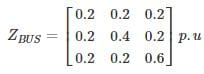If a 3 phase fault occurs at BUS – 2, the p.u. fault current in each phase is – (in pu)

Detailed Solution for Test: Power Systems- 5 - Question 2

Three phase fault current is given by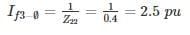Test: Power Systems- 5 - Question 3

### A single line diagram of a power system is shown in the figure. The per unit values of line impedances are given. The sum of diagonal elements of YBUS matrix is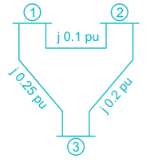Detailed Solution for Test: Power Systems- 5 - Question 3

Z12 = j0.18 pu ⇒ y12 = -j10 pu

Z13 = j0.25 pu ⇒ y13 = -j4 pu

Z23 = j0.2 pu ⇒ y23 = -j5 pu

Y11 = y12 + y13 = -j14 pu

Y22 = y21 + y23 = -j15 pu

Y33 = y31 + y32 = -j9 pu

Sum of diagonal elements = -j14 – j15 – j9

= -j38 pu

*Answer can only contain numeric values
Test: Power Systems- 5 - Question 4

The power system network is having a 60 bus system. The YbusYbus of this power system network is having 70% of sparse. The minimum number of transmission lines that exist in the bus system___

Detailed Solution for Test: Power Systems- 5 - Question 4

Total elements in Ybus = 60 × 60 = 3600

Non – zero = 0.3 × 3600 = 1080

Diagonal = 60

Mutual elements = 660

Transmission lines =1020/2=510

Test: Power Systems- 5 - Question 5

The single line diagram of a power system network having 3 buses and 4 lines is shown in the figure below. The line data is provided in the associated table. The values of the (2, 2) and (2,1) elements of the bus admittance matrix (YBUS) are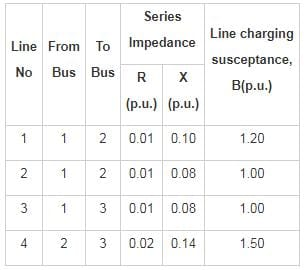Detailed Solution for Test: Power Systems- 5 - Question 5

From the table

Impedance of line 1 = 0.01 + j0.01
Admittance of line 1 =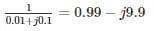Impedance of line 2 = 0.01 + j0.08

Admittance of line 2 =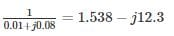Resultant admittance between the bus1 and bus2

y21 = y12 = 0.99 – j9.9 + 1.538 – j 12.3

= 2.528 – j22.2

Impedance of line 3 = 0.01 + j0.08

Admittance of line 3 =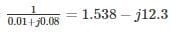y13 = 1.538 – j12.3

Impedance of line 4 = 0.02 + j0.14

Admittance of line 4 =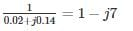Charging susceptance at bus2

2yc2 = sum of charging susceptance of line 1, 2 and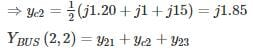= 2.528 - j22.2 + j1.85 + 1 - j7

= 3.528 – j 27.35

YBUS(2,1) = - y21

= - 2.528 + j22.2

*Answer can only contain numeric values
Test: Power Systems- 5 - Question 6

For a Z bus system Z11 = j0.25,Z12 = j0.02,Z13 = j0.05,z14 = j0.04

There are 2 generators at bus 1 and 3 and their sub-transient reactance was induced while calculating Z bus. A three-phase fault occurs at bus 1. The magnitude of pu current supplied by generator 3 whose sub-transient reactance is j0.05 pu is___ (in pu)

Detailed Solution for Test: Power Systems- 5 - Question 6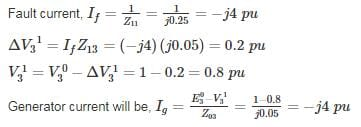Test: Power Systems- 5 - Question 7

The bus admittance matrix for the network shown in the figure is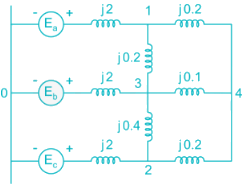Detailed Solution for Test: Power Systems- 5 - Question 7

After converting the given impedances into admittances,

y11 = -j0.5 - j5 - j5 = -j10.5

y22 = -j0.5 - j2.5 - j2.5 = -j5 = -j8.0

y33 = -j0.5 - j5 - j10 - j2.5 = -j18.0

y44 = -j5 - j10 - j5 = -j20.0

y12 = y21 = 0

y13 = y31 = j5.0

y14 = y41 = j5.0

y23 = y32 = j2.5

y24 = y42 = j5.0

y34 = y43 = j10.0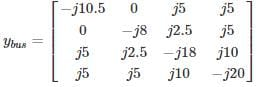Test: Power Systems- 5 - Question 8

Two generators G1 and G2 are connected with cable having a reactance of j3 PU and the load demand at two buses are SD1 = 20 + j20 PU and SD2 = 25 + j2.5 PU the total reactive power in PU at the generating station G1 when δ = 20° is ______ PU

Detailed Solution for Test: Power Systems- 5 - Question 8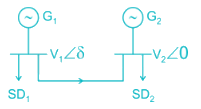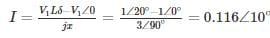S = V1I* = 1∠20° × .116∠-10 = 0.116∠10

S= SD1 + s

= 20 + 20j + .114 + 0.020j

S1 = 20.114 + 20.02 j

Total power = Active power + Reactive power

∴ Reactive power = 20.02 PU

Test: Power Systems- 5 - Question 9

A generator is connected to an infinite bus through a double circuit line as shown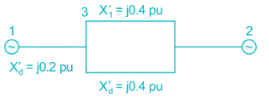The admittance matrix Y is given by

Detailed Solution for Test: Power Systems- 5 - Question 9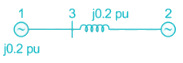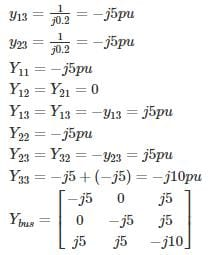Test: Power Systems- 5 - Question 10

The bus impedance matrix of a 4 – bus power system network is given by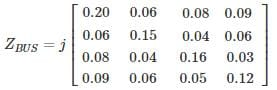An element having an impedance of j 0.12 pu is connected b/n ref bus and bus 2. Calculate new value of Z22.

Detailed Solution for Test: Power Systems- 5 - Question 10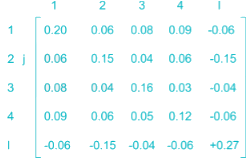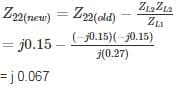## GATE Electrical Engineering (EE) 2023 Mock Test Series

22 docs|274 tests
Information about Test: Power Systems- 5 Page
In this test you can find the Exam questions for Test: Power Systems- 5 solved & explained in the simplest way possible. Besides giving Questions and answers for Test: Power Systems- 5, EduRev gives you an ample number of Online tests for practice

## GATE Electrical Engineering (EE) 2023 Mock Test Series

22 docs|274 tests(Scan QR code)